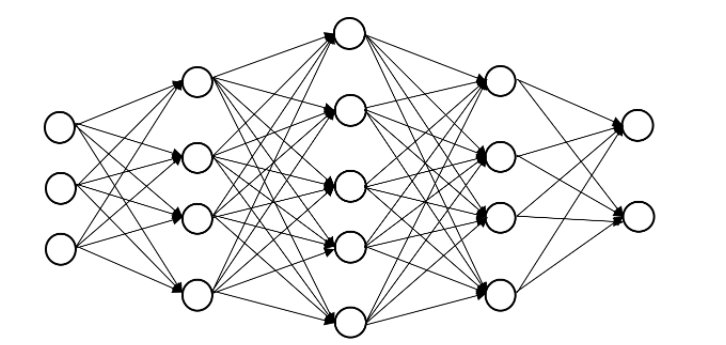Net-informations.com

# Statistics for Data Science

Statistics is the science that concerned with developing and studying methods for collect, organize, analyse and inference of conclusions from quantitative data .

## Types of Statistics

Statistics is divided into two categories:

1. Descriptive statistics
2. Inferential statistics

Descriptive statistics describes the properties of population and sample data and Inferential Statistics which uses those properties to test hypotheses and draw conclusions. Population is a complete set, this means that the entire group that you want to draw conclusions about, while a Sample is a subset of the entire population.

Statistics is a fundamental tool of Data Science because statistics form the basic foundation of all the Machine Learning algorithms. So, it is an important prerequisite for applied Machine Learning, as it helps you to select, evaluate and interpret predictive models .## Machine Learning

Process of solving a problem in Machine Learning with the help of statistics:

1. Define the problem
2. Identify the required data
3. Prepare and pre-process
4. Model the data
5. Train and test
6. Verify and deploy

net-informations.com (C) 2022    Founded by raps mk